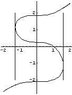# Differential Equations Problem

amcavoy
I have the following problem:

$$y'=\frac{3x^{2}}{3y^{2}-4};\quad y(1)=0$$

I solved this, and came up with $y^{3}-4y-x^{3}=-1$

My problem lies in finding the interval of definition. From the original DE, it is clear that $|y|\neq \frac{2\sqrt{3}}{3}$. Then, after plugging that in for y and solving for what x can't be, I come up with:

$$\left|x^{3}-1\right|\neq \frac{16\sqrt{3}}{9}$$

...however, my reasoning doesn't seem to work here. Apparently, the answer is:

$$\left|x^{3}-1\right|<\frac{16\sqrt{3}}{3}$$

Can someone help me out here?

Thanks a lot.Last edited:

Homework Helper
Gold Member
Dearly Missed
Well, the y-value can't be allowed to slide past the point of singularity when starting on 1, can it?

amcavoy
Alright, well that explains why it is less-than, but why is the denominator 3 instead of 9? Each time I work it out, I come up with 9 in the denominator.

Staff Emeritus
Gold Member
Well, show your work!amcavoy
$$\left|x^{3}-1\right|=y\left(y^{2}-4\right)$$

I then plug in $y=\frac{2\sqrt{3}}{3}$ (which is one of the values y cannot be) to see what value(s) of x would give this:

$$\left|x^{3}-1\right|\neq\frac{2\sqrt{3}}{3}\left(\frac{4}{3}-4\right)$$

..and upon solving for this particular value, I come up with $\left|x^{3}-1\right|\neq\frac{16\sqrt{3}}{9}$, which is incorrect. Why is this?

Thanks again.

Homework Helper
apmcavoy said:
$$\left|x^{3}-1\right|=y\left(y^{2}-4\right)$$

I then plug in $y=\frac{2\sqrt{3}}{3}$ (which is one of the values y cannot be) to see what value(s) of x would give this:

$$\left|x^{3}-1\right|\neq\frac{2\sqrt{3}}{3}\left(\frac{4}{3}-4\right)$$

..and upon solving for this particular value, I come up with $\left|x^{3}-1\right|\neq\frac{16\sqrt{3}}{9}$, which is incorrect. Why is this?

Thanks again.

$$\frac{16}{3\sqrt{3}}$$

is correct. Can you generate an implicit plot of the solution? I've attached one. Do you understand why it looks like that and why the ENTIRE plot satisfies the differential equation even at the points of vertical tangency? I included the two vertical lines to show where the values of x are that you calculated.

#### Attachments

•odeplot1.JPG
3.5 KB · Views: 306
Staff Emeritus
Gold Member
Well, I presume you meant to say:

$$\left|x^{3}-1\right|=\left|y\left(y^{2}-4\right)\right|$$

Anyways, here's a problem:

You know that y cannot be equal to ±2√3/3.

However, that does not mean that y(y²-4) cannot be equal to

(2√3/3)((2√3/3)²-4)

Last edited:
amcavoy
Ok that makes sense. Thank you everyone for your help. By the way, which program did you use to generate that plot? I use Linux and can't find anything that is relatively inexpensive or free for graphing.

Thanks again.

Homework Helper
apmcavoy said:
Ok that makes sense. Thank you everyone for your help. By the way, which program did you use to generate that plot? I use Linux and can't find anything that is relatively inexpensive or free for graphing.

Thanks again.

I used Mathematica but I'm sure others would do. I tell you what, suppose you just absolutely had to write from scratch in C++, a program to plot it. You could do that right? Just for each value of x in the domain, solve the cubic polynomial for y, get the (real) roots, put them all together, and there you go.Staff Emeritus
Gold Member
Bleh, if I had remembered it was a point of tangency, I could have found the other value of y much more easily when x³-1 = 16√3/9.(Oh well, at least I only had to solve a quadratic!)

I used Mathematica but I'm sure others would do. I tell you what, suppose you just absolutely had to write from scratch in C++, a program to plot it. You could do that right? Just for each value of x in the domain, solve the cubic polynomial for y, get the (real) roots, put them all together, and there you go.$$x=\sqrt{1+y^3-4y}$$